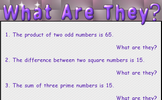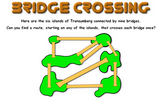# Ratio

### Term 2 starting in week 10 :: Estimated time: 2 weeks

• Using ratio language
• Ratio and fractions
• Introducing the ratio symbol
• Calculating ratio
• Using scale factors
• Calculating scale factors
• Ratio and proportion problems

This page should remember your ticks from one visit to the next for a period of time. It does this by using Local Storage so the information is saved only on the computer you are working on right now.

## Lesson Starters

Here are some suggestions for whole-class, projectable resources which can be used at the beginnings of each lesson in this block.

### 1st Lesson#### Aunty Dotty

A real life situation requiring the division an amount of money according to a given ratio.

### 2nd Lesson#### What are they?

A starter about sums, products, differences, ratios, square and prime numbers.

### 3rd Lesson#### Plant More Trees

If six girls can plant 90 trees in a day. How many trees can ten girls plant in a day? The unitary method.

### 4th Lesson#### Aunt Sophie's Post Office

Work out the number of stamps needed to post a parcel.

### 5th Lesson#### Bridge Crossing

The classic puzzle of finding a route which crosses each bridge once.

### 6th Lesson#### Cars

Calculate the total cost of four cars from the information given.

Some of the Starters above are to reinforce concepts learnt, others are to introduce new ideas while others are on unrelated topics designed for retrieval practice or and opportunity to develop problem-solving skills.

White Rose ResourcesEnd of block assessments provide a quick progress check at the end of each block of learning to make sure students have understood the content covered. This Scheme of Learning was produced by White Rose Maths and is used here with permission granted on 30th June 2021.For All: